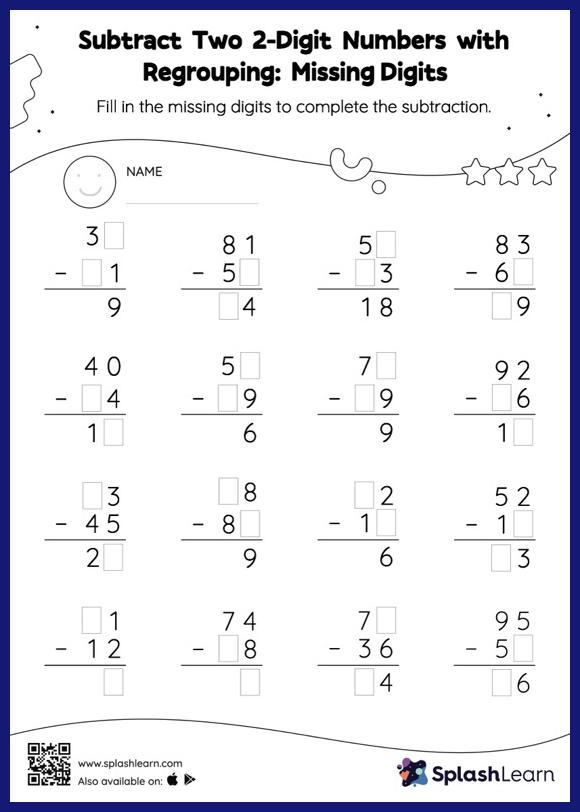# Subtract Two 2-Digit Numbers with Regrouping: Missing Digits Worksheet

Home > Subtract Two 2-Digit Numbers with Regrouping: Missing DigitsHelp your little one develop a knack for math with this subtract two 2-digit numbers with regrouping worksheet. To find the missing number on this worksheet, students can utilize the count back strategy or the connection between addition and subtraction. In subtract two 2-digit numbers with regrouping worksheet, they must additionally regroup numbers to reach the solution. This worksheet is about practicing the column method in which numbers are written one on top of another. In this method, students use their place value understanding to solve the problems.